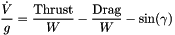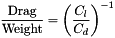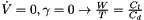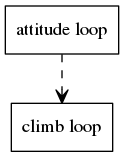Paparazzi UAS  v5.14.0_stable-0-g3f680d1 Paparazzi is a free software Unmanned Aircraft System.
energy_ctrl.c File Reference

Total Energy (speed + height) control for fixed wing vehicles. More...

#include "firmwares/fixedwing/guidance/energy_ctrl.h"
#include "state.h"
#include "firmwares/fixedwing/nav.h"
#include "generated/airframe.h"
#include "autopilot.h"
#include "subsystems/abi.h"Include dependency graph for energy_ctrl.c:

Go to the source code of this file.

## Macros

#define V_CTL_AUTO_GROUNDSPEED_MAX_SUM_ERR   100

#define V_CTL_ALTITUDE_MAX_CLIMB   2;

#define STALL_AIRSPEED   NOMINAL_AIRSPEED

#define V_CTL_GLIDE_RATIO   8.

#define AIRSPEED_SETPOINT_SLEW   1

#define V_CTL_MAX_ACCELERATION   0.5

#define V_CTL_THROTTLE_SLEW   1.

## Functions

static void ac_char_average (float *last_v, float new_v, int count)

static void ac_char_update (float throttle, float pitch, float climb, float accelerate)

static void accel_cb (uint8_t sender_id, uint32_t stamp, struct Int32Vect3 *accel)

static void body_to_imu_cb (uint8_t sender_id, struct FloatQuat *q_b2i_f)

void v_ctl_init (void)

void v_ctl_altitude_loop (void)
outer loop More...

static float low_pass_vdot (float v)

void v_ctl_climb_loop (void)
Auto-throttle inner loop. More...

void v_ctl_throttle_slew (void)
Computes slewed throttle from throttle setpoint called at 20Hz. More...

## Variables

uint8_t v_ctl_mode = V_CTL_MODE_MANUAL

uint8_t v_ctl_climb_mode = V_CTL_CLIMB_MODE_AUTO_THROTTLE

uint8_t v_ctl_auto_throttle_submode = V_CTL_CLIMB_MODE_AUTO_THROTTLE

float v_ctl_auto_throttle_sum_err = 0

float v_ctl_altitude_setpoint
in meters above MSL More...

float v_ctl_altitude_pre_climb
Path Angle. More...

float v_ctl_altitude_pgain

float v_ctl_airspeed_pgain

float v_ctl_altitude_error
in meters, (setpoint - alt) -> positive = too low More...

float v_ctl_max_climb

float v_ctl_max_acceleration

float v_ctl_climb_setpoint

float v_ctl_desired_acceleration

float v_ctl_auto_throttle_cruise_throttle

float v_ctl_auto_throttle_nominal_cruise_throttle

float v_ctl_auto_throttle_nominal_cruise_pitch

float v_ctl_auto_throttle_climb_throttle_increment

float v_ctl_auto_throttle_pitch_of_vz_pgain

float v_ctl_auto_throttle_of_airspeed_pgain

float v_ctl_auto_throttle_of_airspeed_igain

float v_ctl_auto_pitch_of_airspeed_pgain

float v_ctl_auto_pitch_of_airspeed_igain

float v_ctl_auto_pitch_of_airspeed_dgain

float v_ctl_energy_total_pgain

float v_ctl_energy_total_igain

float v_ctl_energy_diff_pgain

float v_ctl_energy_diff_igain

float v_ctl_auto_airspeed_setpoint
in meters per second More...

float v_ctl_auto_airspeed_setpoint_slew

float v_ctl_auto_airspeed_controlled

float v_ctl_auto_groundspeed_setpoint
in meters per second More...

float v_ctl_auto_groundspeed_pgain

float v_ctl_auto_groundspeed_igain

float v_ctl_auto_groundspeed_sum_err

pprz_t v_ctl_throttle_setpoint

pprz_t v_ctl_throttle_slewed

float v_ctl_pitch_setpoint

uint8_t v_ctl_speed_mode

static struct FloatQuat imu_to_body_quat

static struct Int32Vect3 accel_imu_meas

static abi_event accel_ev

static abi_event body_to_imu_ev

float ac_char_climb_pitch = 0.0f

float ac_char_climb_max = 0.0f

int ac_char_climb_count = 0

float ac_char_descend_pitch = 0.0f

float ac_char_descend_max = 0.0f

int ac_char_descend_count = 0

float ac_char_cruise_throttle = 0.0f

float ac_char_cruise_pitch = 0.0f

int ac_char_cruise_count = 0

static const float dt_attidude = 1.0 / ((float)CONTROL_FREQUENCY)

float lp_vdot 

## Detailed Description

Total Energy (speed + height) control for fixed wing vehicles.

Energy:Equilibrium:with:• glide angle:• level flight:Strategy:

• thrust = path + acceleration[g] (total energy)
• pitch = path - acceleration[g] (energy balance)

Pseudo-Control Unit = dimensionless acceleration [g]

- pitch <-> pseudocontrol:    sin(Theta) steers Vdot in [g]
- throttle <-> pseudocontrol: motor characteristic as function of V x throttle steeds VDotDefinition in file energy_ctrl.c.

## Macro Definition Documentation

 #define AIRSPEED_SETPOINT_SLEW   1

Definition at line 158 of file energy_ctrl.c.

Referenced by v_ctl_climb_loop().

 #define STALL_AIRSPEED   NOMINAL_AIRSPEED

Definition at line 151 of file energy_ctrl.c.

Referenced by v_ctl_altitude_loop().

 #define V_CTL_ALTITUDE_MAX_CLIMB   2;

Definition at line 146 of file energy_ctrl.c.

Referenced by potential_task(), v_ctl_altitude_loop(), and v_ctl_init().

 #define V_CTL_AUTO_GROUNDSPEED_MAX_SUM_ERR   100

Definition at line 129 of file energy_ctrl.c.

Referenced by v_ctl_climb_loop().

Definition at line 165 of file energy_ctrl.c.

Referenced by v_ctl_init().

 #define V_CTL_GLIDE_RATIO   8.

Definition at line 154 of file energy_ctrl.c.

Referenced by v_ctl_climb_loop().

 #define V_CTL_MAX_ACCELERATION   0.5

Definition at line 161 of file energy_ctrl.c.

Referenced by v_ctl_init().

 #define V_CTL_THROTTLE_SLEW   1.

Definition at line 447 of file energy_ctrl.c.

Referenced by ArduIMU_init(), and v_ctl_throttle_slew().

## Function Documentation

 static void ac_char_average ( float * last_v, float new_v, int count )
static

Definition at line 180 of file energy_ctrl.c.

Referenced by ac_char_update().Here is the caller graph for this function:

 static void ac_char_update ( float throttle, float pitch, float climb, float accelerate )
static

Definition at line 185 of file energy_ctrl.c.

References ac_char_average(), stateGetSpeedEnu_f(), and Int32Vect3::z.

Referenced by v_ctl_climb_loop().Here is the call graph for this function:Here is the caller graph for this function:

 static void accel_cb ( uint8_t sender_id, uint32_t stamp, struct Int32Vect3 * accel )
static

Definition at line 204 of file energy_ctrl.c.

References accel_imu_meas.

Referenced by v_ctl_init().Here is the caller graph for this function:

 static void body_to_imu_cb ( uint8_t sender_id, struct FloatQuat * q_b2i_f )
static

Definition at line 211 of file energy_ctrl.c.

References float_quat_invert(), and imu_to_body_quat.

Referenced by v_ctl_init().Here is the call graph for this function:Here is the caller graph for this function:

 static float low_pass_vdot ( float v )
static

Definition at line 321 of file energy_ctrl.c.

Referenced by v_ctl_climb_loop().Here is the caller graph for this function:

 void v_ctl_altitude_loop ( void )

outer loop

Computes v_ctl_climb_setpoint and sets v_ctl_auto_throttle_submode

Definition at line 298 of file energy_ctrl.c.Here is the caller graph for this function:

 void v_ctl_climb_loop ( void )

Auto-throttle inner loop.

Definition at line 336 of file energy_ctrl.c.

Referenced by attitude_loop().Here is the caller graph for this function:

 void v_ctl_init ( void )

Definition at line 217 of file energy_ctrl.c.

 void v_ctl_throttle_slew ( void )

Computes slewed throttle from throttle setpoint called at 20Hz.

Computes throttle_slewed from throttle_setpoint.

Definition at line 452 of file energy_ctrl.c.

Referenced by attitude_loop().Here is the caller graph for this function:

## Variable Documentation

 int ac_char_climb_count = 0

Definition at line 172 of file energy_ctrl.c.

 float ac_char_climb_max = 0.0f

Definition at line 171 of file energy_ctrl.c.

 float ac_char_climb_pitch = 0.0f

Definition at line 170 of file energy_ctrl.c.

 int ac_char_cruise_count = 0

Definition at line 178 of file energy_ctrl.c.

 float ac_char_cruise_pitch = 0.0f

Definition at line 177 of file energy_ctrl.c.

 float ac_char_cruise_throttle = 0.0f

Definition at line 176 of file energy_ctrl.c.

 int ac_char_descend_count = 0

Definition at line 175 of file energy_ctrl.c.

 float ac_char_descend_max = 0.0f

Definition at line 174 of file energy_ctrl.c.

 float ac_char_descend_pitch = 0.0f

Definition at line 173 of file energy_ctrl.c.

 abi_event accel_ev
static

Definition at line 140 of file energy_ctrl.c.

 struct Int32Vect3 accel_imu_meas
static

Definition at line 138 of file energy_ctrl.c.

Referenced by accel_cb(), and v_ctl_climb_loop().

 abi_event body_to_imu_ev
static

Definition at line 141 of file energy_ctrl.c.

 const float dt_attidude = 1.0 / ((float)CONTROL_FREQUENCY)
static

Definition at line 290 of file energy_ctrl.c.

Referenced by v_ctl_climb_loop().

static

Definition at line 291 of file energy_ctrl.c.

 struct FloatQuat imu_to_body_quat
static

Definition at line 137 of file energy_ctrl.c.

Referenced by body_to_imu_cb(), v_ctl_climb_loop(), and v_ctl_init().

 float lp_vdot

Definition at line 318 of file energy_ctrl.c.

 float v_ctl_airspeed_pgain

Definition at line 91 of file energy_ctrl.c.

Referenced by v_ctl_climb_loop(), and v_ctl_init().

 float v_ctl_altitude_error

in meters, (setpoint - alt) -> positive = too low

Definition at line 92 of file energy_ctrl.c.

Referenced by h_ctl_course_loop(), and v_ctl_altitude_loop().

 float v_ctl_altitude_pgain

Definition at line 90 of file energy_ctrl.c.

Referenced by v_ctl_altitude_loop(), and v_ctl_init().

 float v_ctl_altitude_pre_climb

Path Angle.

Definition at line 89 of file energy_ctrl.c.

Referenced by v_ctl_altitude_loop(), and v_ctl_init().

 float v_ctl_altitude_setpoint

in meters above MSL

Definition at line 88 of file energy_ctrl.c.

 float v_ctl_auto_airspeed_controlled

Definition at line 123 of file energy_ctrl.c.

Referenced by send_airspeed(), v_ctl_climb_loop(), and v_ctl_init().

 float v_ctl_auto_airspeed_setpoint

in meters per second

Definition at line 121 of file energy_ctrl.c.

 float v_ctl_auto_airspeed_setpoint_slew

Definition at line 122 of file energy_ctrl.c.

Referenced by v_ctl_climb_loop(), and v_ctl_init().

 float v_ctl_auto_groundspeed_igain

Definition at line 127 of file energy_ctrl.c.

Referenced by v_ctl_climb_loop(), and v_ctl_init().

 float v_ctl_auto_groundspeed_pgain

Definition at line 126 of file energy_ctrl.c.

Referenced by v_ctl_climb_loop(), and v_ctl_init().

 float v_ctl_auto_groundspeed_setpoint

in meters per second

Definition at line 125 of file energy_ctrl.c.

Referenced by send_airspeed(), v_ctl_climb_loop(), and v_ctl_init().

 float v_ctl_auto_groundspeed_sum_err

Definition at line 128 of file energy_ctrl.c.

Referenced by v_ctl_climb_loop(), and v_ctl_init().

 float v_ctl_auto_pitch_of_airspeed_dgain

Definition at line 113 of file energy_ctrl.c.

Referenced by v_ctl_climb_loop(), and v_ctl_init().

 float v_ctl_auto_pitch_of_airspeed_igain

Definition at line 112 of file energy_ctrl.c.

Referenced by v_ctl_climb_loop(), and v_ctl_init().

 float v_ctl_auto_pitch_of_airspeed_pgain

Definition at line 111 of file energy_ctrl.c.

Referenced by v_ctl_climb_loop(), and v_ctl_init().

 float v_ctl_auto_throttle_climb_throttle_increment

Definition at line 106 of file energy_ctrl.c.

Referenced by v_ctl_climb_loop(), and v_ctl_init().

 float v_ctl_auto_throttle_cruise_throttle

Definition at line 103 of file energy_ctrl.c.

Referenced by formation_flight(), potential_task(), start_formation(), and stop_formation().

 float v_ctl_auto_throttle_nominal_cruise_pitch

Definition at line 105 of file energy_ctrl.c.

Referenced by v_ctl_climb_loop(), and v_ctl_init().

 float v_ctl_auto_throttle_nominal_cruise_throttle

Definition at line 104 of file energy_ctrl.c.

Referenced by v_ctl_climb_loop(), and v_ctl_init().

 float v_ctl_auto_throttle_of_airspeed_igain

Definition at line 110 of file energy_ctrl.c.

Referenced by v_ctl_climb_loop(), and v_ctl_init().

 float v_ctl_auto_throttle_of_airspeed_pgain

Definition at line 109 of file energy_ctrl.c.

Referenced by v_ctl_climb_loop(), and v_ctl_init().

 float v_ctl_auto_throttle_pitch_of_vz_pgain

Definition at line 107 of file energy_ctrl.c.

Referenced by v_ctl_climb_loop(), and v_ctl_init().

 uint8_t v_ctl_auto_throttle_submode = V_CTL_CLIMB_MODE_AUTO_THROTTLE

Definition at line 76 of file energy_ctrl.c.

Referenced by h_ctl_course_loop(), nav_periodic_task(), and send_calibration().

 float v_ctl_auto_throttle_sum_err = 0

Definition at line 77 of file energy_ctrl.c.

Referenced by send_calibration().

 uint8_t v_ctl_climb_mode = V_CTL_CLIMB_MODE_AUTO_THROTTLE

Definition at line 75 of file energy_ctrl.c.

 float v_ctl_climb_setpoint

Definition at line 98 of file energy_ctrl.c.

Referenced by send_desired(), v_ctl_altitude_loop(), v_ctl_climb_loop(), and v_ctl_init().

 float v_ctl_desired_acceleration

Definition at line 101 of file energy_ctrl.c.

Referenced by v_ctl_climb_loop().

 float v_ctl_energy_diff_igain

Definition at line 119 of file energy_ctrl.c.

Referenced by v_ctl_climb_loop(), and v_ctl_init().

 float v_ctl_energy_diff_pgain

Definition at line 118 of file energy_ctrl.c.

Referenced by v_ctl_climb_loop(), and v_ctl_init().

 float v_ctl_energy_total_igain

Definition at line 116 of file energy_ctrl.c.

Referenced by v_ctl_climb_loop(), and v_ctl_init().

 float v_ctl_energy_total_pgain

Definition at line 115 of file energy_ctrl.c.

Referenced by v_ctl_climb_loop(), and v_ctl_init().

 float v_ctl_max_acceleration

Definition at line 95 of file energy_ctrl.c.

Referenced by v_ctl_climb_loop(), and v_ctl_init().

 float v_ctl_max_climb

Definition at line 94 of file energy_ctrl.c.

Referenced by v_ctl_altitude_loop(), and v_ctl_init().

 uint8_t v_ctl_mode = V_CTL_MODE_MANUAL

Definition at line 74 of file energy_ctrl.c.

 float v_ctl_pitch_setpoint

Definition at line 133 of file energy_ctrl.c.

Referenced by attitude_loop(), h_ctl_guidance_loop(), and v_ctl_climb_loop().

 uint8_t v_ctl_speed_mode

Definition at line 135 of file energy_ctrl.c.

Referenced by v_ctl_init().

 pprz_t v_ctl_throttle_setpoint

Definition at line 131 of file energy_ctrl.c.

 pprz_t v_ctl_throttle_slewed

Definition at line 132 of file energy_ctrl.c.

Referenced by attitude_loop(), send_bat(), and v_ctl_throttle_slew().